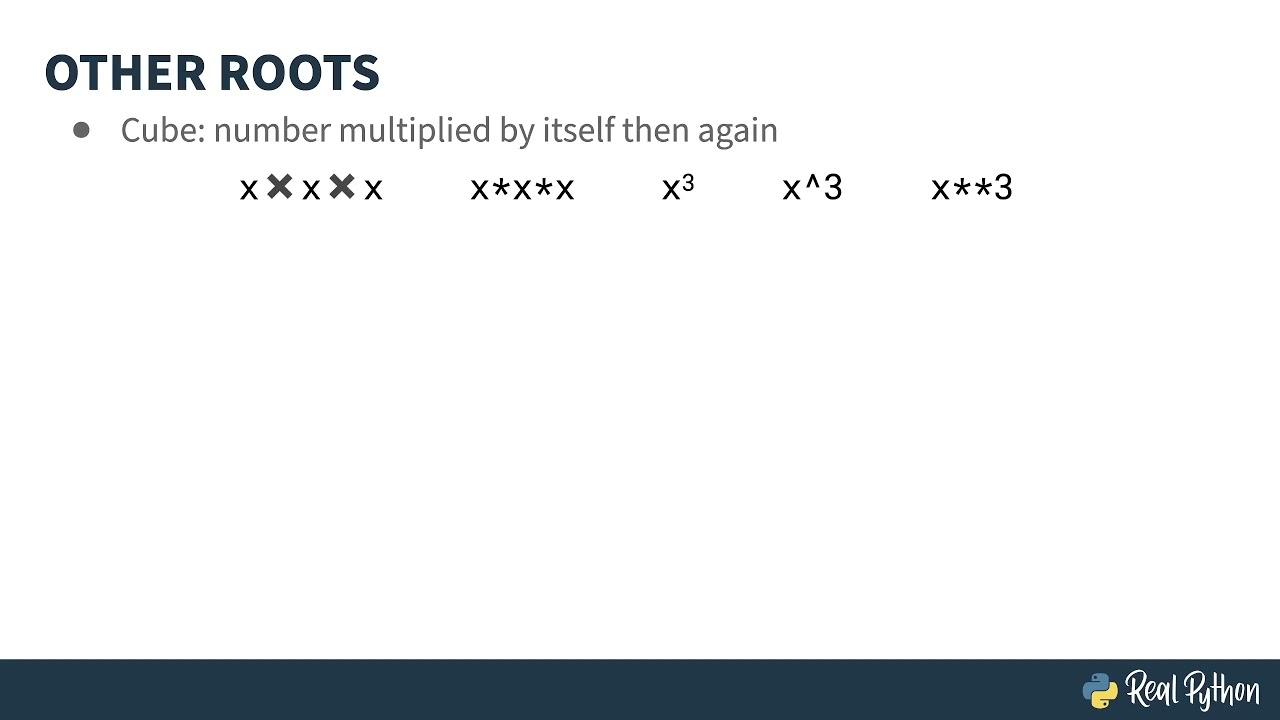1626400505

# Using Square Root in Python

Are you trying to solve a quadratic equation? Maybe you need to calculate the length of one side of a right triangle. You can use the math module’s sqrt() method for determining the square root of a number. This video covers the use of math.sqrt() as well as related methods.